# Mathematical Modeling of Closed Loop Pulsating Heat Pipe by Using Artificial Neural Networks

Mathematical Modeling of Closed Loop Pulsating Heat Pipe by Using Artificial Neural Networks
Institute of Aeronautical Engineering, Dundigal, Hyderabad, Telangana 500043, India

RVR and JC College of Engineering, Mechanical Engineering, Guntur 522019, Andhra Pradesh, India

JNTUH, Mechanical Engineering, Hyderabad 500085, Telangana, India

Corresponding Author Email:
n.santhisree@iare.ac.in
Page:
955-962
|
DOI:
https://doi.org/10.18280/ijht.390332
2 October 2020
|
Accepted:
10 April 2021
|
Published:
30 June 2021
| Citation

OPEN ACCESS

Abstract:

Pulsating heat pipe is one of the prominent technology for thermal management of electronic devices. It consists of three sections namely evaporator, adiabatic and condenser section. PHP is a two phase passive device having efficient and quick ability of transferring heat from evaporator section to condenser section. At first an 8 turn pulsating heat pipe of closed loop ends (CLPHP) with copper tube capillary dimensions is investigated experimentally for different fill ratios and for different inclinations by varying range of heat inputs. Different working fluids viz Water, Acetone, Ethanol and Methanol are considered for the experimentation. One of the recent analytical technology for modelling of CLPHPs is Artificial Neural Network (ANN) approach. The analytical models are having limited scope of applicability and they are simple in nature. The present paper describes Validation of experimental data by training prediction model ANN with available data. Three input nodes such as input heat, fill ratio and angle of inclination and one output node corresponding to PHP that is thermal resistance are considered. The feed forward neural network (FFNN) architecture is adopted for predictions. By using the physical phenomena of the system modelling are clearly known for obtaining feasible results which is main function of ANN. The predicted data validates experimental data in a satisfactory range and the results are found to be in good agreement with in the range of ± 10 percent.

Keywords:

angle of inclination, ANN model, CLPHP, fill ratio (FR), heat input, thermal resistance (Rth), working fluid

1. Introduction

Pulsating heat pipes are mainly developed for control of electronic devices heat dissipation rates. It’s a novel wickless heat pipe with phase change phenomena and invented by Akachi and Polášek .. PHP consists of Evaporator where heat input is supplied to the device, adiabatic section and condenser sections at which the vapour condenses and becomes liquid i.e. rejection of heat take place. By pulsating motion heat transfer takes place from one end to other end. Surface tension and gravitational forces plays an important role in the working mechanism of heat transfer. In order to reduce the force due to surface tension over the force due to gravitation, the diameter of the tube is selected as capillary dimensions. Due to capillary dimensions the fluid distributes itself in the tube. This causes liquid slugs and vapour bubbles in the tube. As the physical phenomena occurs in PHP are complex, the application and scope of analytical models are limited in nature. The operating mechanism of PHP is mainly depending on physical, geometrical and operational parameters which influences its performance. Simulation studies on pulsating heat pipes were conducted by Jokar et al. . And natural algorithms for simulation was applied. This simulator clearly explains complex behaviour of the PHP by using nonlinear structure. Numerical model to investigate the pressure losses in the tube with respect to number of turns was developed by Sakulchangsatjatai et al. , and Mameli et al. . In this, the correlations of heat transfer are developed for predicting the PHP performance. Using ANN and regression analysis, Prediction models for pulsating heat pipe were conducted by Patel and Mehta . All the literature data related to experiments and 9 variables are considered as input to develop prediction models are collected and developed Feed-forward back-propagation model to verify the data. On chaotic flow numerical investigations are carried out by Pouryoussefi and Zhang . For analysis evaporative heating and condenser cooling constant wall temperatures are applied. Fully connected feed forward multilayer ANN is used to train with test data and then experimental data is validated by Khandekar et al. . ANN consists of two input nodes and single output node. The results concluded that if sufficient data is available ANN approach seems to be a promising approach. Various nonlinear models based on fluctuations in the wall temperatures to understand the complex flow distribution in the PHP are implemented by Qu et al. , and Song and Xu . A neural network of two layers to predict the PHP behaviour is studied by Shokouhmand et al. . The results obtained are appropriate for predicting effective parameters trend on performance of PHP and they are in concurrent with available data. To predict the thermal performance of a PHP, Han et al.  carried out experiments. The thermal performance of PHP is influenced by operational parameters (heat flux applied, PHP orientation and fill ratio), inner diameter, number of turns as geometrical parameters and pure fluids, binary mixtures and nano fluids are as working fluids. The correlation for start-up heat flux and start-up mechanism of PHP is theoretically described by Qu and Ma . The results explains that the influence of working fluids makes the PHP’s internal heat and mass transfer phenomena more complex.

From the literature it is observed that closed loop pulsating heat pipe prediction models are developed with limited fluids at a specified fill ratio and orientation only. The main objective of the present work is to carry out a comprehensive work on water, ethanol, methanol and acetone fluids with 50%, 60% and 75% fill ratios at 0-degree, 45-degree and 90-degree positions. The experimental values are compared with prediction values. The feed forward neural network is used over other methods because in this method only in one direction that is in the forward direction the information always moves. The connections between the nodes do not form a cycle that is it does not go backwards. The transfer of nodes is from input to output nodes through the hidden nodes.

2. Artificial Neural Networks

To understand the pulsating motion of liquid slugs and vapour bubbles of fluid in tube and also effect of parameters influence on CLPHP many prediction models are developed. But the number of parameters prediction is very much limited. To predict the thermal performance of PHP the correlations of heat transfer mechanism including all the parameters are so far not available. Keeping this in view to develop prediction model for thermal performance of PHP the present research work is carried out by using ANN approach. ANN approach is one of the modelling techniques used to predict the data where the understanding of mechanism in a device is difficult.

The design and functioning of human brain and nervous system is the main motivation for the design of ANN device. It’s a data-driven machine learning tool. It’s neither an algorithm nor actual hardware. All the processing elements are interconnected and makes different layers. These layers are useful to obtain a relationship between inputs and outputs, learning and memorizing the data. Usually, the structure of ANN consists of three layers shown in Figure 1. For receiving data input layer, for processing hidden layer and to send computed information an output layer. The artificial neurons are represented by nodes of each layer. Through weights the interconnection between the nodes and its preceding layer is possible. To match with the required output through the learning process by adjusting of associated weights the desired output is obtained. To model complex functions all, the individual neurons are connected with each other. The output vector is obtained when input vector is accepted by network.

## 1.png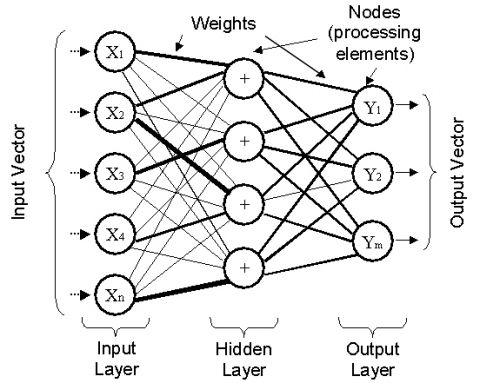Figure 1. Architecture of artificial neural network 

By ANN always it is not possible to get prediction of new data. This is due to overtraining the data. To avoid this data is set into different parts. For training process almost 70% of data is assigned. In the remaining 30%, 15% of data us used for testing and the remaining 15% is for validation. For developing accurate model more data is required. Therefore, training data is used for this purpose. To train the network the learning algorithm is used. The algorithm measures actual and desired output vector difference. The error resulted uses an iterative method for calculating weights and to improve network such a way to reduce error. Several times this process is repeated till the error is in the required level so that it becomes valid model for prediction. To reduce the error to check generalization and accuracy the validation data is used. The final behaviour of network is examined by validating data.

3. The Parameters Influencing CLPHP Performance

The experimental work is carried out on an 8 turn closed loop pulsating heat pipe made up of copper. Copper tube with 2 mm and 3.1 mm capillary dimensions and of length 264 mm was bent into 8 U-turns into major section of CLPHP such as evaporator, adiabatic and condenser section. The evaporator box of size 330X90X55 mm are placed at the bottom and the condenser of size 320X95X55 mm are placed at the top to insert the parallel tubes. The CLPHP performance is mainly depending upon the parameters like working fluid, volume fill ratio, inclination angle, number of turns, inner diameter of the tube, heat input supplied etc. Fill ratio is defined as the ratio of liquid volume to the total volume of PHP. It varies from 0% to 100% i.e. CLPHP tubes without any fluid (bare tubes) or empty device means no fluid in the tube is referred as 0% filling. In this case the heat transfer in CLPHP is only by pure conduction mode. A fully filled tube refers to 100% filling ratio. In this case there is no pulsating effect in the tube. Due to buoyancy the liquid circulates in the tube and buoyancy helps for heat transfer. Therefore, between 10% and 90% fill ratios only CLPHP can function in a pulsating mode. The exact value of fill ratio is mainly depending upon working fluids, construction of PHP and other parameters that effect PHP. Different mass flow rates are maintained for different heat inputs. The mass flow rate depends upon the properties such as its specific heat, density etc. of working fluid considered.

Heat is supplied at evaporator section of CLPHP. The liquid converts into vapour at evaporator and gets condensed in the condenser section. Amount of heat supplied is mainly depending upon the type of fluid used and its boiling point. The orientation of CLPHP also another important parameter which effects the performance. Different inclinations varying from 0 degree to 180 degrees is possible. In all these cases the physics that is underlined for understanding of heat transfer mechanism is not so clear and is differs with respect to its operation.

The schematic diagram of an 8 turn CLPHP experimental setup is as shown in Figure 2. The experiment is conducted for working fluids namely Water, Ethanol, Methanol and Acetone. By incorporating thermocouples at evaporator and condenser sections, the temperatures were recorded and heat input is supplied at bottom end of evaporator section by using variac. The ratio of difference in temperature with its heat input gives the performance parameter thermal resistance, the required output.

## 2.png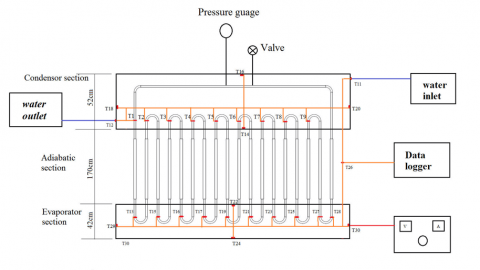Figure 2. Schematic of experimental setup

The experimental results are noted for all the working fluids considered at different fill ratios (50%, 60% and 75%) and also at different orientations (0-degree, 45-degrees and 90-degree). These results are validated by using ANN.

To develop the Artificial Neural Network (ANN) model a neural network toolbox in MATLAB was used This model was developed by considering Heat input (20-100 W) depending upon the working fluid, Fill ratio (50%, 60% and 75%) and inclination angle (0-degree, 45-degrees and 90-degree) as input parameters and thermal resistance as output parameter. The following Tables 1-4 gives different process parameters considered for different working fluids namely Water, Acetone, Ethanol and Methanol (Considered for the present study).

Table 1. Process parameters and their levels for water CLPHP

 Process parameters Levels 1 2 3 Heat Input 20 60 100 Fill Ratio (%) 50 60 75 Inclination angle 0 45 90

Table 2. Process parameters and their levels for acetone CLPHP

 Process parameters Levels 1 2 3 Heat Input 20 40 60 Fill Ratio (%) 50 60 75 Inclination angle 0 45 90

Table 3. Process parameters and their levels for ethanol CLPHP

 Process parameters Levels 1 2 3 Heat Input 20 40 60 Fill Ratio (%) 50 60 75 Inclination angle 0 45 90

Table 4. Process parameters and their levels for methanol CLPHP

 Process parameters Levels 1 2 3 Heat Input 20 40 60 Fill Ratio (%) 50 60 75 Inclination angle 0 45 90

Initially, into the neural network the input data were fed and then to achieve the output it was preceded for simulation in Figure 3. By using 33 factorial designs simulations are carried out taking into account of maximum and minimum limits of the fluid considered. A total of 27 experiments investigations were considered to predict the data using ANN architecture namely Feed Forward Neural Network (FFNN).

## 3.pngFigure 3. Feed forward back propagation ANN

The Tables 5a and 5b describes the experimental plan for CLPHP modeling. Different operating parameters that affect the CLPHP performance are considered in different combinations. The combination includes heat input, fill ratio and angle of inclination for different fluids Water, Acetone, Ethanol and Methanol.

Table 5a. Experimental plan for modeling water and acetone CLPHP

 Water Acetone Q FR Angle Q FR Angle 20 50 0 60 50 0 60 50 0 60 50 45 100 50 0 60 50 90 20 50 45 60 60 0 60 50 45 60 75 0 100 50 45 60 60 45 20 50 90 60 60 90 60 50 90 60 75 45 100 50 90 60 75 90 20 60 0 40 50 0 60 60 0 40 50 45 100 60 0 40 60 0 20 60 45 40 50 90 60 60 45 40 60 45 100 60 45 40 75 0 20 60 90 40 60 90 60 60 90 40 75 45 100 60 90 40 75 90 20 75 0 20 50 0 60 75 0 20 60 0 100 75 0 20 50 45 20 75 45 20 75 0 60 75 45 20 60 45 100 75 45 20 50 90 20 75 90 20 60 90 60 75 90 20 75 45 100 75 90 20 75 90

Table 5b. Experimental plan for modeling ethanol and methanol CLPHP

 Ethanol Methanol Q FR Angle Q FR Angle 60 50 0 60 75 45 60 50 45 60 50 0 60 50 90 60 50 45 60 60 0 60 50 90 60 60 45 60 60 0 60 75 0 60 75 0 60 75 45 60 60 45 60 75 90 60 60 90 60 60 90 60 75 90 40 50 0 40 50 0 40 50 45 40 50 45 40 50 90 40 60 0 40 75 0 40 50 90 40 60 0 40 60 45 40 75 90 40 60 90 40 75 45 40 75 0 40 60 45 40 75 45 40 60 90 40 75 90 20 50 0 20 50 0 20 50 45 20 60 0 20 60 0 20 75 0 20 60 45 20 50 45 20 75 0 20 60 45 20 60 90 20 50 90 20 50 90 20 60 90 20 75 45 20 75 45 20 75 90 20 75 90
4. Procedure for Executing ANN Model

By using TRAINLM and LEARNGDM the training and Adaption learning functions are considered as shown in Figure 4.

## 4.pngFigure 4. Creating network using ANN

## 5.png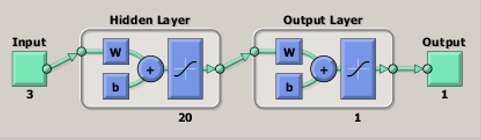Figure 5. Neural network training

For every neural network model the following model is adopted.

• For input and output data excel data sheet has to be prepared as shown in table.
• In the workspace of MATLAB the data has to be imported. While importing the heading should not be imported, only data to be imported.
• With a suitable number of neurons and layers proper network, input target data and training performance are selected.
• As per chosen parameters hit create button for development of new network.
• By selecting the Train network button for the given input and output Train the network. The following Figure 5 explains the training of neural network.
• W refers to weights and b refers to biases in hidden layer of network. The input layer passes these data to the first hidden layer. The weighted input parameters (weight 9 input parameter) and bias are processed through a transfer function in this layer.
• Predicted result and error are generated by selecting simulating button.
• Export the data obtained in to workspace.
• Post processing can be done by pasting the exported data in to excel.
5. Results and Discussions

The output thermal resistance is determined by using experimental values for all considerations adopted for experimentation. These resistance values are validated by using ANN. The error can be calculated as percentage error by using the following formula.

Error (%) =$\frac{(\text { Rth }(\text { prediction })-\text { Rth }(\text { Experimental }))}{\text { Rth(Experimental) }}$

5.1 For working fluid water

The following Figures 6-8 show the comparison of water at different fill ratio and at different inclinations by considering both ANN and Experimental result.

5.1.1 At 50% fill ratio

The percentage error observed for all the readings regarding CLPHP operating with working fluid water when operating at 50%FR at different inclination angles was below ±5% range.

## 6a.png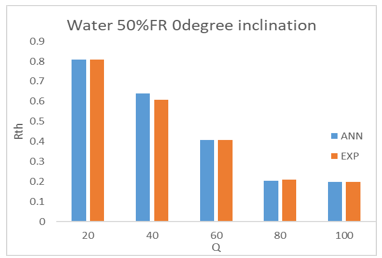## 6b.png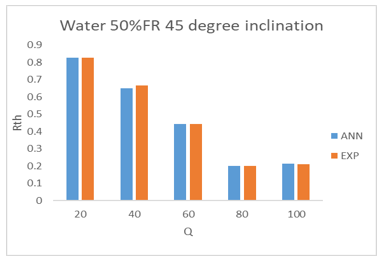## 6c.png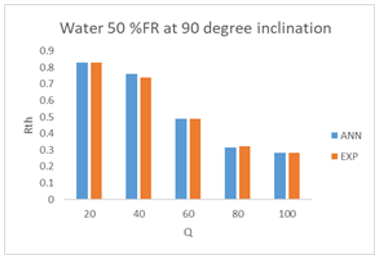Figure 6. Comparison of experimental Rth with predicted ANN at 50% FR and 0,45,90-degree inclinations water CLPHP

5.1.2 At 60% fill ratio

The percentage error observed for all the readings regarding PHP operating with working fluid water when operating at 60%FR at different inclination angles was below ±10% range.

## 7a.png## 7b.png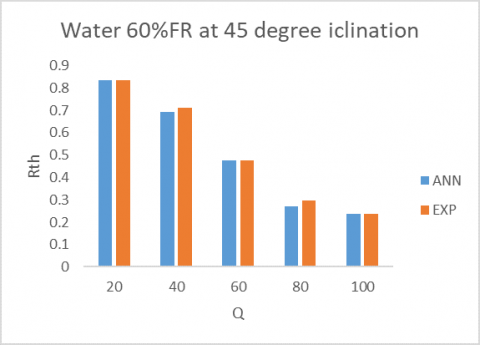## 7c.png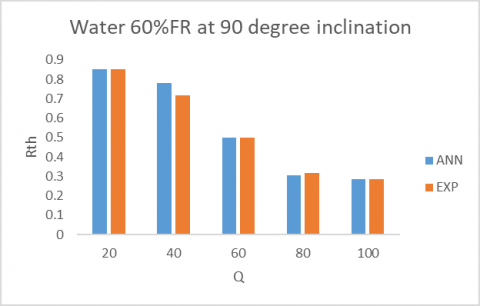Figure 7. Comparison of experimental Rth with predicted ANN at 60% FR and 0,45,90-degree inclinations for water CLPHP

5.1.3 At 75% fill ratio

The percentage error observed for all the readings regarding PHP operating with working fluid water when operating at 75%FR at different inclination angles was below ±13% range. The following graph Figure 8 shows these variation.

Among all the working conditions of CLPHP with water, when it is operating at 50% fill ratio is having an error of less value i.e ±5% compared with 60% and 75% fill ratios.Thermal resistance value are high for higher filling ratio compared to lower filling ratio. At low heat transfer limit, higher thermal resistance is observed and performance of CLPHP is very poor. For high filling ratios, sufficient bubbles are not developed to provide pumping action in the fluid. So at this FR drastic deterioration of performance occurs.The formation of bubbles will results in better heat transfer rates and hence performnce improvement.

## 8a.png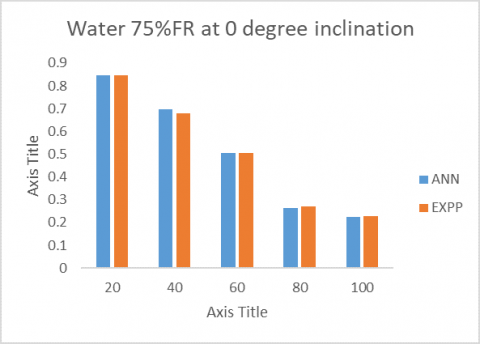## 8b.png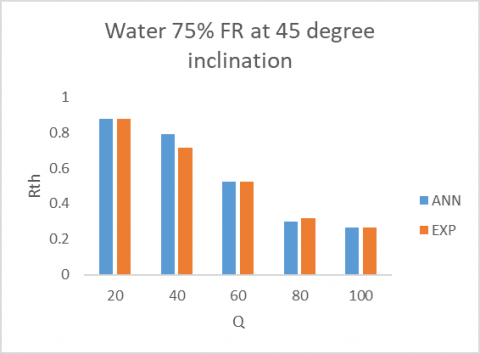## 8c.png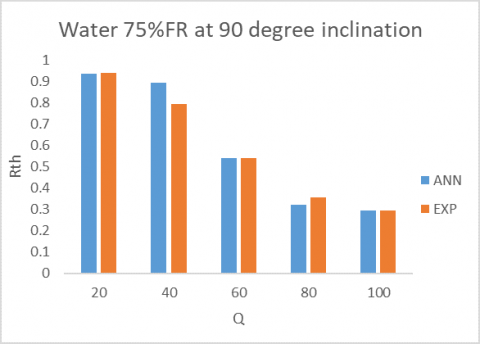Figure 8. Comparison of experimental Rth with predicted ANN at 75% FR and 0,45,90-degree inclinations for water CLPHP

5.2 For working fluid acetone

Acetone has low value of latent heat.At a given temperature and presure quck evaporation of liquid takes place has it has low latent heat value i.e 520.56 kJ/kg.The velocities of liquid slugs wil be increased and results in improvement of heat ransfer.The physical and chemical properties of fluid are: boilin point is 56.2℃, density 748.5 kg/m3 in liqud form, specific heat 2.28 kJ/kg-K in liquid form, 1.385 kJ/kg-K for vapor acetone and its density is 19.09X10-3 N/m. The following Figure 9 shows comparison of Acetone fluid at different fill ratios and at different inclination angles by considering experimental results and ANN predictions.

When the CLPHP with acetone as working fluid was operating at 50% fill ratio and at 0-degree inclination the error obtained was ±6% and for 45-degree and 90-degree the percentage of errors are ±1% and ±1% respectively.

When the CLPHP with working fluid acetone was operating at 60% fill ratio and at 0-degree inclination the error obtained was ±2% and for 45-degree and 90 degree the percentage of errors are ±3% and ±5% respectively as shown in Figure 10.

## 9.png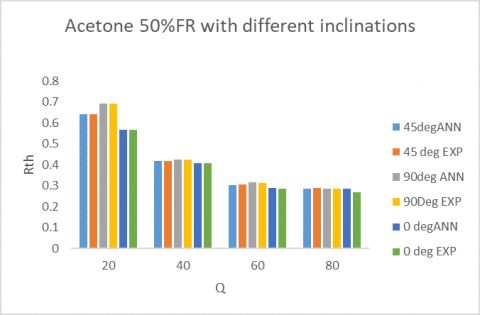Figure 9. Comparison of experimental Rth with predicted ANN at 50% FR and 0,45,90-degree inclinations for acetone CLPHP

## 10.pngFigure 10. Comparison of experimental Rth with predicted ANN at 60% FR and 0,45,90-degree inclinations for acetone CLPHP

## 11.pngFigure 11. Comparison of experimental Rth with predicted ANN value at 75% FR and 0,45,90-degree inclinations for acetone CLPHP

When CLPHP with working fluid acetone was operating at 75% fill ratio and at 0-degree inclination the error obtained was ±9%. where as for 45-degree and 90 degree the percentage of errors are ±1% and ±6% respectively shown in Figure 11. And observed that 5% error is observed for acetone flud at 60% fill ratio and 90-degree inclination position.

5.3 For working fluid ethanol

The following set of graphs (Figures 12, 13 and 14) shows the comparative analysis of experimentally obtained thermal resistance values with ANN predictions values obtained by training in nntool for PHP working with ethanol as working fluid. The heat input is considered in the range 20-80 watts and fill ratios considered are 50%, 60% and 75% at inclinations of 0-degree, 45-degree and 90-degree positions.

## 12.png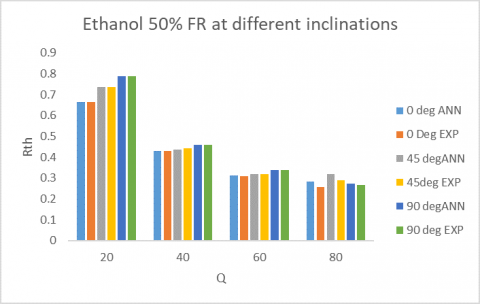Figure 12. Comparison of experimental Rth with predicted ANN at 50% FR and 0,45,90-degree inclinations for ethanol CLPHP

When CLPHP is operating with Ethanol working fluid at 50% filling ratio, (i) at 0-degree position the error in thermal resistance obtained is ±10% (ii) at 45-degree position inclination the error is ±11% (iii) and at an inclination angle 90-degree it was observed as ±3%.

## 13.pngFigure 13. Comparison of experimental Rth with predicted ANN at 60% FR and 0,45,90-degree inclinations for ethanol CLPHP

When CLPHP is operating with Ethanol working fluid at 60% filling ratio, (i) at 0-degree position the error in thermal resistance obtained is ±3% (ii) at 45-degree position inclination the error is ±8% (iii) and at an inclination angle 90-degree it was observed as ±9%.

## 14.pngFigure 14. Comparison of experimental Rth with predicted ANN at 75% FR and 0,45,90-degree inclinations for ethanol CLPHP

When CLPHP is operating with Ethanol working fluid at 75% filling ratio, (i) at 0-degree position the error in thermal resistance obtained is ±2% (ii) at 45-degree position inclination the error is ±1% (iii) and at an inclination angle 90-degree it was observed as ±3%.

5.4 For working fluid methanol

The experiments are carried out for CLPHP by considering methanol as working fluid. The temperatures of evaporator and condenser at different locations are recorded by using data logger and hence thermal resistance was found by varying heat input. Fill ratios of 50%, 60% and 75% are considered. All the experiments are carried at 0 –degree, 45-degree and 90-degree inclinations of PHP. The results obtained are validated with ANN tool. The following group of curves (Figures 15, 16 and 17) represents the variations of Rth in both experimental values and also predicted ANN values.

## 15.png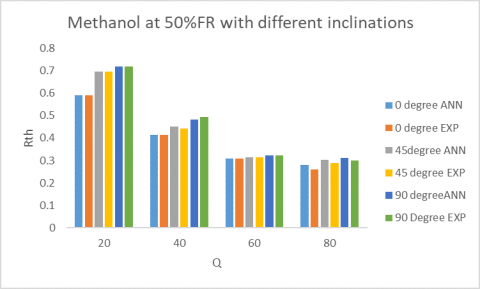Figure 15. Comparison of experimental Rth with predicted ANN at 50% FR and 0,45,90-degree inclinations for methanol CLPHP

When methanol CLPHP is considered at 50% fill ratio the error percentage obtained experimentally in comparison with predicted ANN thermal resistance was different from one position to other position. At 0-degree inclination position the error is in the range of ±8% whereas for 45 and 90-degree positions it is in the range of ±4% only. This is due to the gravity that acts in 0-degree position.

## 16.png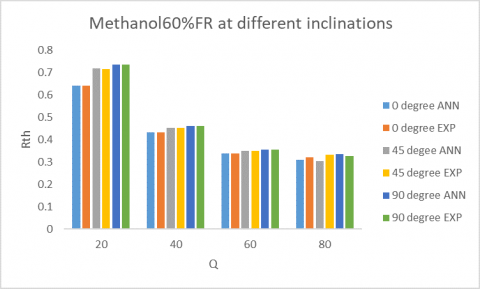Figure 16. Comparison of experimental Rth with predicted ANN Rth at 60% FR and 0,45,90-degree inclinations for methanol CLPHP

For CLPHP operating at 60% fill ratio the error percentage of thermal resistance in comparison with experimental and predicted ANN values is at 0-degree and 90–degree inclination positions ±3% and at 45-degree position observed as ±9%.

## 17.png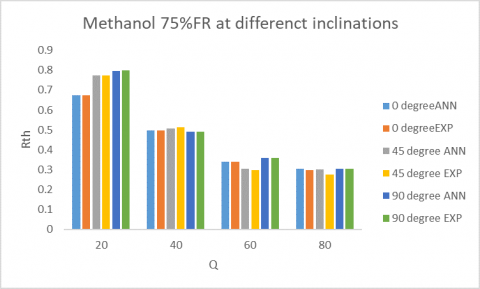Figure 17. Comparison of experimental Rth with predicted ANN at 75% FR and 0,45,90-degree inclinations for methanol CLPHP

For CLPHP operating at 75% fill ratio the error percentage of thermal resistance obtained in comparison with experimental and predicted ANN values is at 0-degree±2%, at 45-degree ±9%. And at 90 -degrees it is 0.2 percent.

6. Conclusions
• For an 8 turn closed loop pulsating heat pipe with four different working fluids viz, water, acetone, ethanol and methanol at different heat inputs a feed forward back propagation algorithm is trained, validated and also used for predicting the data.
• The influencing parameters of CLPHP such as heat input, filling ratio, angle of inclinations is used as input data and thermal resistance as output data is used for ANN modelling (The feed forward neural network (FFNN) architecture is adopted for predictions).
• In case of thermal resistance value based on predicting the output data using ANN, the experimental deviations for Water is maximum of ±13% at different heat inputs, different fill ration and also different orientations. For acetone it is ±9%, whereas for ethanol and methanol is ±10%.
• Among all the working fluids acetone shows least thermal resistance value and also least error value.
• Water shows higher value of thermal resistance compared to other fluids.
• Among all the working fluids considered such as water, acetone, ethanol and methanol at 50%, 60 % and 75% fill ratios operating at 0-degree, 45-degree and 90-degree inclination positions the maximum resistance is observed for water fluid operating at 75% fill ratio and 90-degree inclination position, whereas minimum resistance is observed for acetone at 50% fill ratio and 0-degree inclination position.
• Optimization of parameters that effects performance of CLPHP and the design of it can be made easier with the help of ANN model.
• ANN modelling of CLPHP can be used in practical applications like radiators of automobiles, refrigeration systems and cooling of fuel cells.
References

 Akachi, H., Polášek, F. (1996). Pulsating Heat Pipes. In: Heat Pipe Symposium, Melbourne, Australia, 208-217.

 Jokar, A., Godarzi, A.A., Saber, M., Shafii, M.B. (2016). Simulation and optimization of a pulsating heat pipe using artificial neural network and genetic algorithm. Heat and Mass Transfer, 52(11): 2437-2445. https://doi.org/10.1007/s00231-016-1759-8

 Sakulchangsatjatai, P., Terdtoon, P., Wongratanaphisan, T., Kamonpet, P., Murakami, M. (2004). Operation modeling of closed-end and closed-loop oscillating heat pipes at normal operating condition. Applied Thermal Engineering, 24(7): 995-1008. https://doi.org/10.1016/j.applthermaleng.2003.11.006

 Mameli, M., Marengo, M., Zinna, S. (2012). Numerical model of a multi-turn closed loop pulsating heat pipe: Effects of the local pressure losses due to meanderings. International Journal of Heat and Mass Transfer, 55(4): 1036-1047. https://doi.org/10.1016/j.ijheatmasstransfer.2011.10.006

 Patel, V.M., Mehta, H.B. (2016). Artificial neural network modeling of a closed loop pulsating heat pipe. World Academy of Science, Engineering, and Technology-International Journal of Mechanical, Aerospace, Industrial, Mechatronics, and Manufacturing Engineering, 10: 1754-1757.

 Pouryoussefi, S.M., Zhang, Y. (2016). Numerical investigation of chaotic flow in a 2D closed-loop pulsating heat pipe. Applied Thermal Engineering, 98: 617-627. https://doi.org/10.1016/j.applthermaleng.2015.12.097

 Khandekar, S., Cui, X., Groll, M. (2002). Thermal performance modeling of pulsating heat pipes by artificial neural network. In Proceedings of 12th International Heat Pipe Conference, pp. 215-219.

 Qu, J., Wu, H., Cheng, P., Wang, X. (2009). Non-linear analyses of temperature oscillations in a closed-loop pulsating heat pipe. International Journal of Heat and Mass Transfer, 52(15-16): 3481-3489. https://doi.org/10.1016/j.ijheatmasstransfer.2009.03.012

 Song, Y.X., Xu, J.L. (2009). Chaotic behaviour of pulsating heat pipes. International Journal of Heat and Mass Transfer, 52(13-14): 2932-2941. https://doi.org/10.1016/j.ijheatmasstransfer.2009.02.030

 Shokouhmand, H., Gharib, N., Bahrami, H. (2006). Modeling of closed loop pulsating heat pipes by neural networks. Engineering Systems Design and Analysis, 42487: 131-136. https://doi.org/10.1115/ESDA2006-95417

 Han, X., Wang, X., Zheng, H., Xu, X., Chen, G. (2016). Review of the development of pulsating heat pipe for heat dissipation. Renewable and Sustainable Energy Reviews, 59: 692-709. https://doi.org/10.1016/j.rser.2015.12.350

 Qu, W., Ma, H.B. (2007). Theoretical analysis of startup of a pulsating heat pipe. International Journal of Heat and Mass Transfer, 50(11-12): 2309-2316. https://doi.org/10.1016/j.ijheatmasstransfer.2006.10.043